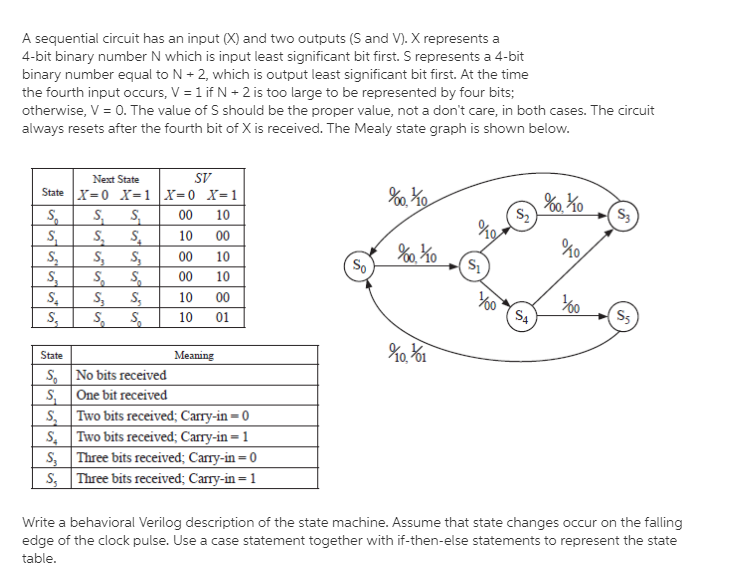A sequential circuit has an input (X) and two outputs (S and V). X represents a4-bit binary number N which is input least significant bit first. S represents a 4-bitbinary number equal to N +2, which is output least significant bit first. At the timethe fourth input occurs, V-1 if N +2 is too large to be represented by four bits;otherwise, V = O. The value of S should be the proper value, not a don't care, in both cases. The circuitalways resets after the fourth bit of X is received. The Mealy state graph is shown belovwNext StateSV00 1010 0000 1000 1010 0010 01KoMeaningNo bits receivedS One bit receivedS, Two bits received, Carry-in-0S, Two bits received, Carry-in-1Three bits received; Carry-in-0S Three bits received, Carry-in -1Write a behavioral Velog description of the state machine. Assume that state changes occur on the fallingedge of the clock pulse. Use a case statement together with if-then-else statements to represent the statetable

Questionhelp_outlineImage TranscriptioncloseA sequential circuit has an input (X) and two outputs (S and V). X represents a 4-bit binary number N which is input least significant bit first. S represents a 4-bit binary number equal to N +2, which is output least significant bit first. At the time the fourth input occurs, V-1 if N +2 is too large to be represented by four bits; otherwise, V = O. The value of S should be the proper value, not a don't care, in both cases. The circuit always resets after the fourth bit of X is received. The Mealy state graph is shown belovw Next State SV 00 10 10 00 00 10 00 10 10 00 10 01 Ko Meaning No bits received S One bit received S, Two bits received, Carry-in-0 S, Two bits received, Carry-in-1 Three bits received; Carry-in-0 S Three bits received, Carry-in -1 Write a behavioral Velog description of the state machine. Assume that state changes occur on the falling edge of the clock pulse. Use a case statement together with if-then-else statements to represent the state table fullscreen
Step 1

Solution:

The following code is described about the behavioral Verilog description for given state graph. That is,

• The code is written using the if-then-else statement.
• State change occurs on the falling edge of the clock pulse is nothing but transition takes place at “negedge”.
• That is, we are always@ (negedge clk).
• Check whether the case is “S0”.
• If the current state is “S1”, then set the “nstate” as “S1” and “Sv” as “10”. Otherwise, set the “nstate” as “S1” and “Sv” as “00”.
• Check whether the case is “S1”.
• If the current state is “S1”, then set the “nstate” as “S4” and “Sv” as “00”. Otherwise, set the “nstate” as “S2” and “Sv” as “10”.
• Check whether the case is “S2”.
• If the current state is “S1”, then set the “nstate” as “S3” and “Sv” as “10”. Otherwise, set the “ns...

Want to see the full answer?

See Solution

Want to see this answer and more?

Our solutions are written by experts, many with advanced degrees, and available 24/7

See Solution
Tagged in

Other